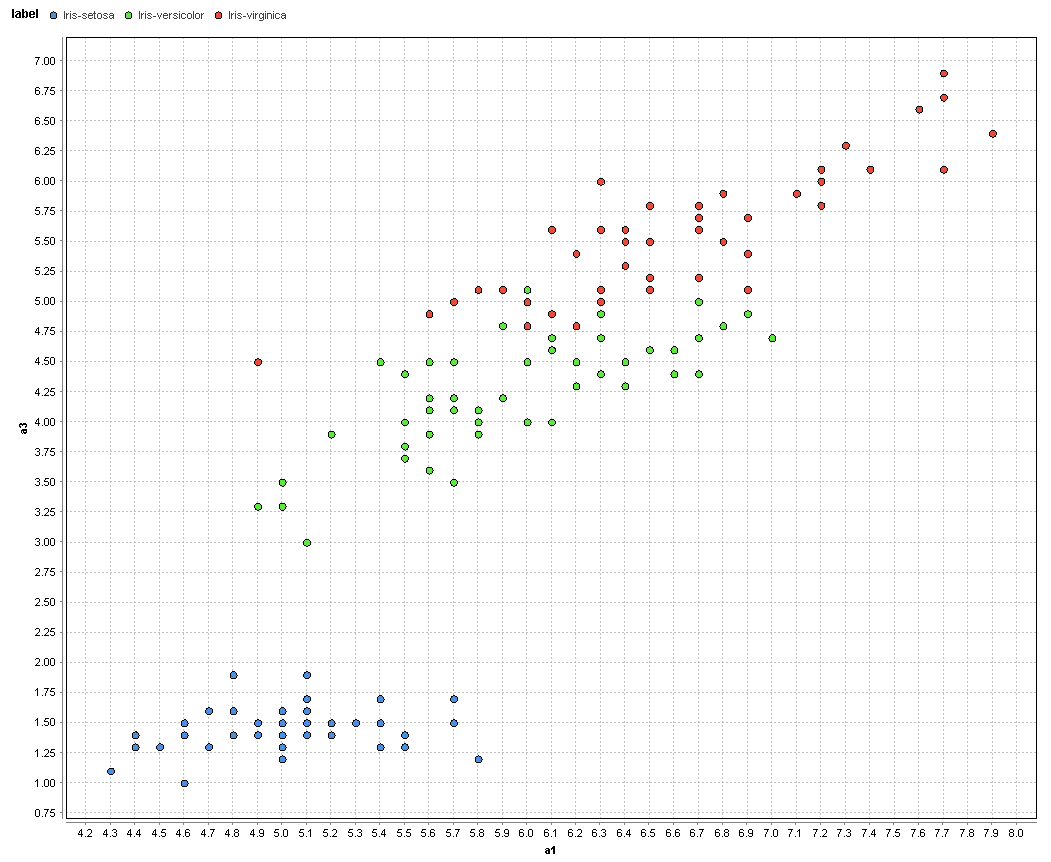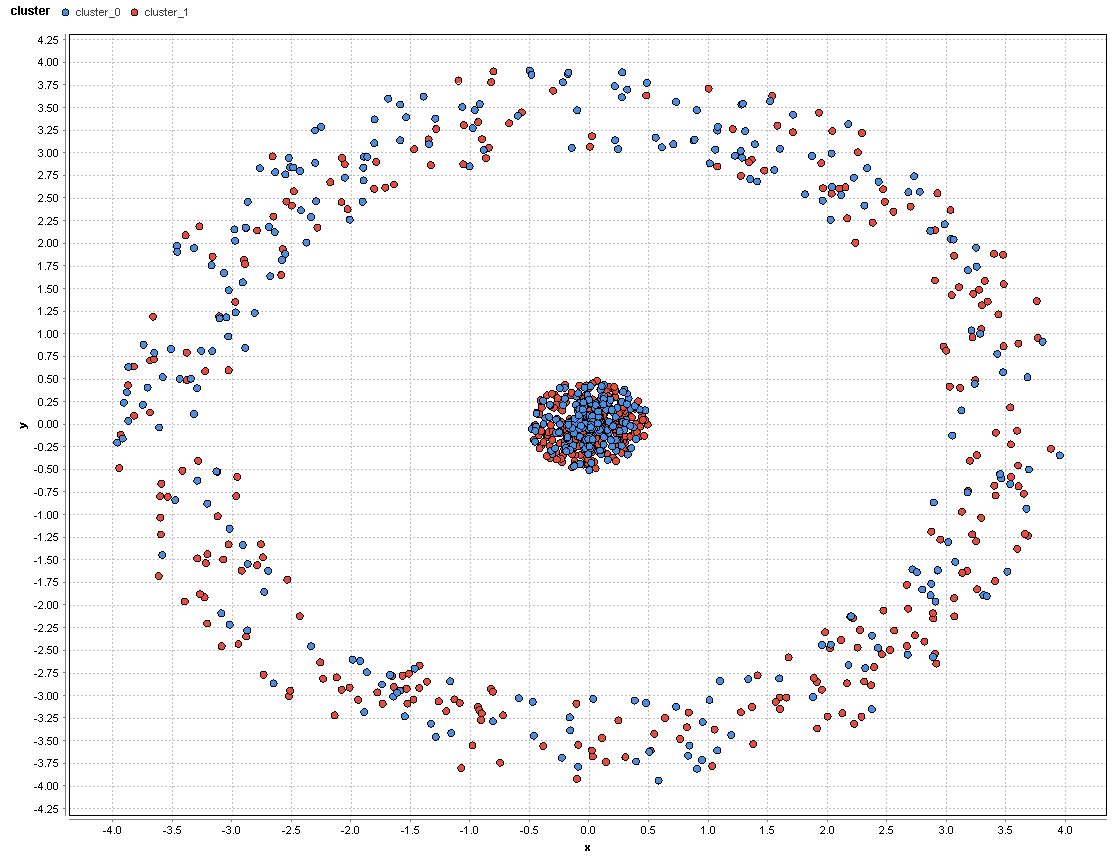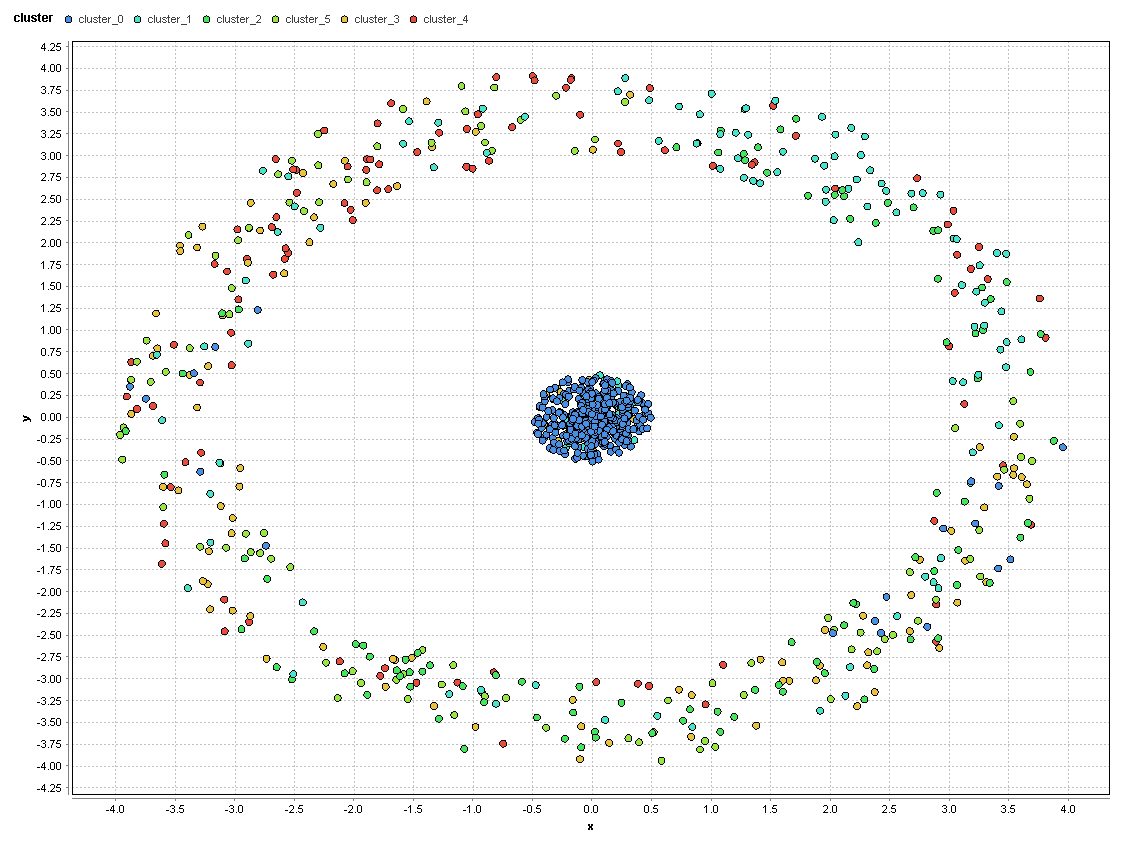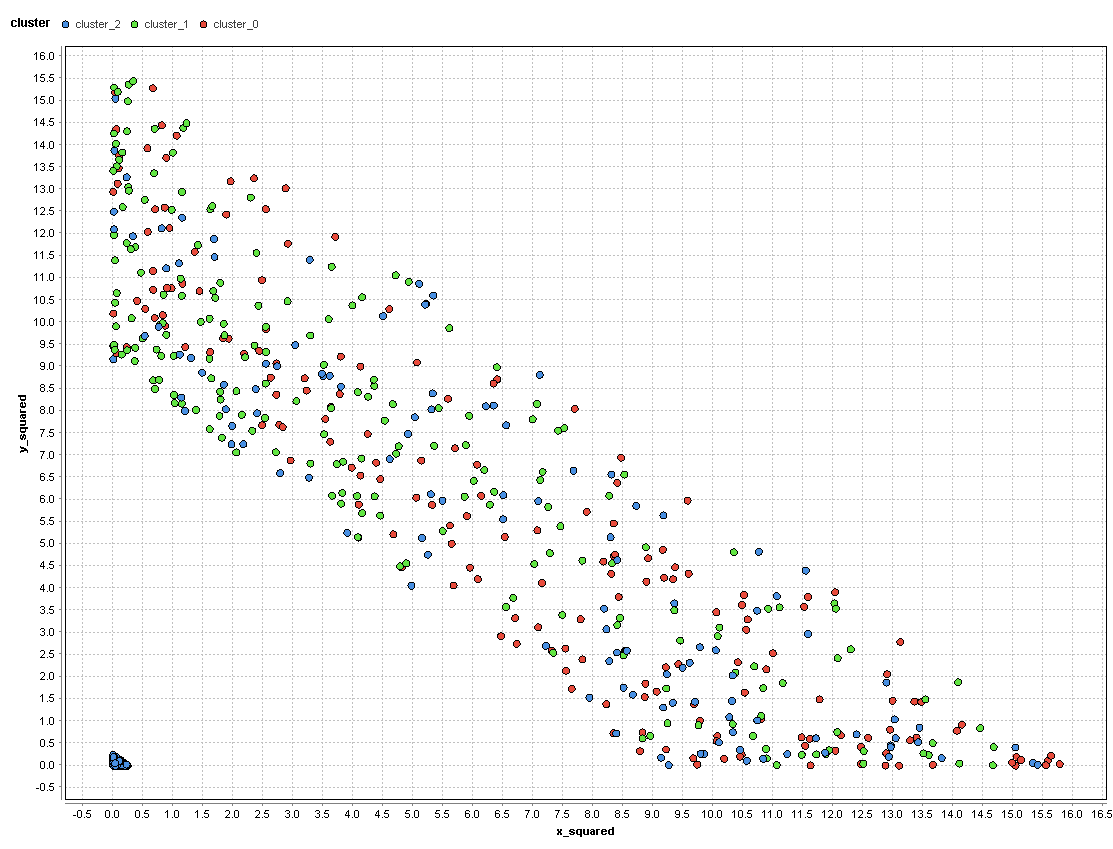# "Kmeans clustering"

Member Posts: 15Contributor I
edited May 2019 in Help
I have the data in the attached csv file. I have to use kmeans for grouping them. I have to make a graph and say how many groups are formed. We have to comment the performance of kmeans and to suggest a better solution. Any ideas???
Tagged:

• Moderator, RapidMiner Certified Analyst, Member Posts: 1,195Unicorn
Hi @bookitsa,

Difficult to represent your dataset(s) if you work in high dimensionnal space (number of attribute = N).
But you can always represent Attribute i vs Attribute j and in color the class of the label and see if some groups appear...
for example here 2 attributes of the Iris dataset (we see that there are 3 groups):If you don't know a priori the number of groups (number of clusters) you can try the 2 following models :
- DBSCAN
- X-Means

Hope it helps,

Regards,

Lionel

• Member Posts: 15Contributor I
In my example above data.csv how many clusters appear? I can not distinquish...and how we find the  number of clusters that appear in a specific data set?
• Member Posts: 15Contributor I
Any ideas?
• Moderator, RapidMiner Certified Analyst, RapidMiner Certified Expert, Member Posts: 1,635Unicorn
There is no right answer to the question "how many clusters appear" for several reasons, but most fundamentally because it is unsupervised vs supervised machine learning.  So there is no "pre-established" truth that is being used to train the algorithm at the individual example level.  Some clustering techniques require you to specify the number of clusters you want to see in advance, such as k-means and its variants.  Other techniques may use an algorithm to determine the best number of clusters, but that will be from the perspective of that particular approach, which may or may not be suited to your problem.  So what is the purpose of the project?  Is there a number of clusters that you expect to find?
If you really have no idea where to start, you might want to try the X-Means operator which will use the k-means approach and use many different values for k and choose the one that best satisfies some statistical measures of fit.  At least you could use that as a starting point.
Brian T.
Lindon Ventures
Data Science Consulting from Certified RapidMiner Experts
• Member Posts: 15Contributor I
The purpose of the project is to use the kmeans in the attached data.csv to make a graph and to say how many groups we believe that are formed. Then to propose a better solution...
• Moderator, RapidMiner Certified Analyst, RapidMiner Certified Expert, Member Posts: 1,635Unicorn
edited January 2019
Did you try the X-Means operator as suggested?  That will give you one recommended value of k that you can work with further.
Brian T.
Lindon Ventures
Data Science Consulting from Certified RapidMiner Experts
• Member Posts: 15Contributor I
Well I tried the Xmeans and this is the xml code. The x-means comes with the minimum of k=3 and all the data are in a circle and some are in the center of the circle. I tried with k=5,10,20 but nothing changes. The only that changes is the color of the clusters and the number. I don' know from that I saw what is the proper number of clusters. Also I have to comment the performance of kmeans and to propose something better(an other algorithm)...

<?xml version="1.0" encoding="UTF-8"?><process version="9.0.003">
<context>
<input/>
<output/>
<macros/>
</context>
<operator activated="true" class="process" compatibility="9.0.003" expanded="true" name="Process">
<process expanded="true">
<operator activated="true" class="retrieve" compatibility="9.0.003" expanded="true" height="68" name="Retrieve data2" width="90" x="45" y="34">
<parameter key="repository_entry" value="//Local Repository/data2"/>
</operator>
<operator activated="true" class="x_means" compatibility="9.0.003" expanded="true" height="82" name="X-Means" width="90" x="246" y="34"/>
<connect from_op="Retrieve data2" from_port="output" to_op="X-Means" to_port="example set"/>
<connect from_op="X-Means" from_port="cluster model" to_port="result 1"/>
<connect from_op="X-Means" from_port="clustered set" to_port="result 2"/>
<portSpacing port="source_input 1" spacing="0"/>
<portSpacing port="sink_result 1" spacing="0"/>
<portSpacing port="sink_result 2" spacing="0"/>
<portSpacing port="sink_result 3" spacing="0"/>
</process>
</operator>
</process>

• Moderator, RapidMiner Certified Analyst, Member Posts: 1,195Unicorn
Hi @bookitsa,

1. I played with your data and I propose humbly an example of presentation using k-Means algorithm :

When you graphically represent your data, we can conclude "visually" that there are 2 clusters (the center and the circle) :To find a "better solution", you have first to define a performance metrics for your clusters. We can take the Davies Bouldin which mesure the "quality" of your clusters.This is an internal evaluation scheme, where the validation of how well the clustering has been done is made using quantities and features inherent to the dataset.
In this first case (k = 2), we obtain Davies Bouldin = -0,836
Now, to find a better solution, you can find an other "k". You can find this "better value" by using the Optimize Parameters operator (with a search range of k of [2,8]) :
RM concludes k = 6 and Davies Bouldin = 0,570 => That's much better...! :Now to go further, a "better solution" means maybe a "better data preparation",
We can for example generate the attributes X and Y with  :
X = x*x
Y = y*y
We can relaunch the optimizing process with these new features and we obtain :
k = 3 and Davies Bouldin = 0,457 => That's better...! :
and if we represent graphically these news features, we obtain :Hope these elements help...
The process :
<?xml version="1.0" encoding="UTF-8"?><process version="9.1.000">
<context>
<input/>
<output/>
<macros/>
</context>
<operator activated="true" class="process" compatibility="9.1.000" expanded="true" name="Process">
<parameter key="logverbosity" value="init"/>
<parameter key="random_seed" value="2001"/>
<parameter key="send_mail" value="never"/>
<parameter key="process_duration_for_mail" value="30"/>
<parameter key="encoding" value="SYSTEM"/>
<process expanded="true">
<parameter key="csv_file" value="C:\Users\Lionel\Documents\Formations_DataScience\Rapidminer\Tests_Rapidminer\Clustering\data.csv"/>
<parameter key="column_separators" value=","/>
<parameter key="trim_lines" value="false"/>
<parameter key="use_quotes" value="true"/>
<parameter key="quotes_character" value="&quot;"/>
<parameter key="escape_character" value="\"/>
<parameter key="comment_characters" value="#"/>
<parameter key="starting_row" value="1"/>
<parameter key="parse_numbers" value="true"/>
<parameter key="decimal_character" value="."/>
<parameter key="grouped_digits" value="false"/>
<parameter key="grouping_character" value=","/>
<parameter key="infinity_representation" value=""/>
<parameter key="date_format" value=""/>
<parameter key="first_row_as_names" value="true"/>
<list key="annotations"/>
<parameter key="time_zone" value="SYSTEM"/>
<parameter key="locale" value="English (United States)"/>
<parameter key="encoding" value="windows-1252"/>
<list key="data_set_meta_data_information">
<parameter key="0" value="x.true.real.attribute"/>
<parameter key="1" value="y.true.real.attribute"/>
</list>
<parameter key="datamanagement" value="double_array"/>
<parameter key="data_management" value="auto"/>
</operator>
<operator activated="true" class="generate_attributes" compatibility="9.1.000" expanded="true" height="82" name="Generate Attributes" width="90" x="246" y="85">
<list key="function_descriptions">
<parameter key="x_squared" value="x*x"/>
<parameter key="y_squared" value="y*y"/>
</list>
<parameter key="keep_all" value="true"/>
</operator>
<operator activated="true" class="generate_id" compatibility="9.1.000" expanded="true" height="82" name="Generate ID" width="90" x="380" y="85">
<parameter key="create_nominal_ids" value="false"/>
<parameter key="offset" value="0"/>
</operator>
<operator activated="true" class="select_attributes" compatibility="9.1.000" expanded="true" height="82" name="Select Attributes" width="90" x="514" y="85">
<parameter key="attribute_filter_type" value="subset"/>
<parameter key="attribute" value=""/>
<parameter key="attributes" value="x_squared|y_squared"/>
<parameter key="use_except_expression" value="false"/>
<parameter key="value_type" value="attribute_value"/>
<parameter key="use_value_type_exception" value="false"/>
<parameter key="except_value_type" value="time"/>
<parameter key="block_type" value="attribute_block"/>
<parameter key="use_block_type_exception" value="false"/>
<parameter key="except_block_type" value="value_matrix_row_start"/>
<parameter key="invert_selection" value="false"/>
<parameter key="include_special_attributes" value="false"/>
</operator>
<operator activated="true" class="concurrency:optimize_parameters_grid" compatibility="9.1.000" expanded="true" height="145" name="Optimize Parameters (Grid)" width="90" x="648" y="85">
<list key="parameters">
<parameter key="Clustering.k" value="[2.0;8;20;linear]"/>
</list>
<parameter key="error_handling" value="fail on error"/>
<parameter key="log_performance" value="true"/>
<parameter key="log_all_criteria" value="false"/>
<parameter key="synchronize" value="false"/>
<parameter key="enable_parallel_execution" value="true"/>
<process expanded="true">
<operator activated="true" class="concurrency:cross_validation" compatibility="9.1.000" expanded="true" height="145" name="Cross Validation" width="90" x="380" y="85">
<parameter key="split_on_batch_attribute" value="false"/>
<parameter key="leave_one_out" value="false"/>
<parameter key="number_of_folds" value="10"/>
<parameter key="sampling_type" value="automatic"/>
<parameter key="use_local_random_seed" value="false"/>
<parameter key="local_random_seed" value="1992"/>
<parameter key="enable_parallel_execution" value="true"/>
<process expanded="true">
<operator activated="true" class="concurrency:k_means" compatibility="9.1.000" expanded="true" height="82" name="Clustering" width="90" x="179" y="34">
<parameter key="remove_unlabeled" value="false"/>
<parameter key="k" value="2"/>
<parameter key="max_runs" value="10"/>
<parameter key="determine_good_start_values" value="true"/>
<parameter key="measure_types" value="BregmanDivergences"/>
<parameter key="mixed_measure" value="MixedEuclideanDistance"/>
<parameter key="nominal_measure" value="NominalDistance"/>
<parameter key="numerical_measure" value="EuclideanDistance"/>
<parameter key="divergence" value="SquaredEuclideanDistance"/>
<parameter key="kernel_gamma" value="1.0"/>
<parameter key="kernel_sigma1" value="1.0"/>
<parameter key="kernel_sigma2" value="0.0"/>
<parameter key="kernel_sigma3" value="2.0"/>
<parameter key="kernel_degree" value="3.0"/>
<parameter key="kernel_shift" value="1.0"/>
<parameter key="kernel_a" value="1.0"/>
<parameter key="kernel_b" value="0.0"/>
<parameter key="max_optimization_steps" value="100"/>
<parameter key="use_local_random_seed" value="false"/>
<parameter key="local_random_seed" value="1992"/>
</operator>
<connect from_port="training set" to_op="Clustering" to_port="example set"/>
<connect from_op="Clustering" from_port="cluster model" to_port="model"/>
<portSpacing port="source_training set" spacing="0"/>
<portSpacing port="sink_model" spacing="0"/>
<portSpacing port="sink_through 1" spacing="0"/>
</process>
<process expanded="true">
<operator activated="true" class="apply_model" compatibility="9.1.000" expanded="true" height="82" name="Apply Model" width="90" x="45" y="34">
<list key="application_parameters"/>
<parameter key="create_view" value="false"/>
</operator>
<operator activated="true" class="cluster_distance_performance" compatibility="9.1.000" expanded="true" height="103" name="Performance" width="90" x="246" y="34">
<parameter key="main_criterion" value="Davies Bouldin"/>
<parameter key="main_criterion_only" value="false"/>
<parameter key="normalize" value="false"/>
<parameter key="maximize" value="false"/>
</operator>
<operator activated="false" class="data_to_similarity" compatibility="9.1.000" expanded="true" height="82" name="Data to Similarity" width="90" x="179" y="187">
<parameter key="measure_types" value="MixedMeasures"/>
<parameter key="mixed_measure" value="MixedEuclideanDistance"/>
<parameter key="nominal_measure" value="NominalDistance"/>
<parameter key="numerical_measure" value="EuclideanDistance"/>
<parameter key="divergence" value="GeneralizedIDivergence"/>
<parameter key="kernel_gamma" value="1.0"/>
<parameter key="kernel_sigma1" value="1.0"/>
<parameter key="kernel_sigma2" value="0.0"/>
<parameter key="kernel_sigma3" value="2.0"/>
<parameter key="kernel_degree" value="3.0"/>
<parameter key="kernel_shift" value="1.0"/>
<parameter key="kernel_a" value="1.0"/>
<parameter key="kernel_b" value="0.0"/>
</operator>
<connect from_port="model" to_op="Apply Model" to_port="model"/>
<connect from_port="test set" to_op="Apply Model" to_port="unlabelled data"/>
<connect from_op="Apply Model" from_port="labelled data" to_op="Performance" to_port="example set"/>
<connect from_op="Apply Model" from_port="model" to_op="Performance" to_port="cluster model"/>
<connect from_op="Performance" from_port="performance" to_port="performance 1"/>
<connect from_op="Performance" from_port="example set" to_port="test set results"/>
<portSpacing port="source_model" spacing="0"/>
<portSpacing port="source_test set" spacing="0"/>
<portSpacing port="source_through 1" spacing="0"/>
<portSpacing port="sink_test set results" spacing="0"/>
<portSpacing port="sink_performance 1" spacing="0"/>
<portSpacing port="sink_performance 2" spacing="0"/>
</process>
</operator>
<connect from_port="input 1" to_op="Cross Validation" to_port="example set"/>
<connect from_op="Cross Validation" from_port="model" to_port="model"/>
<connect from_op="Cross Validation" from_port="test result set" to_port="output 1"/>
<connect from_op="Cross Validation" from_port="performance 1" to_port="performance"/>
<portSpacing port="source_input 1" spacing="0"/>
<portSpacing port="source_input 2" spacing="0"/>
<portSpacing port="sink_performance" spacing="0"/>
<portSpacing port="sink_model" spacing="0"/>
<portSpacing port="sink_output 1" spacing="0"/>
<portSpacing port="sink_output 2" spacing="0"/>
</process>
</operator>
<connect from_op="Read CSV" from_port="output" to_op="Generate Attributes" to_port="example set input"/>
<connect from_op="Generate Attributes" from_port="example set output" to_op="Generate ID" to_port="example set input"/>
<connect from_op="Generate ID" from_port="example set output" to_op="Select Attributes" to_port="example set input"/>
<connect from_op="Select Attributes" from_port="example set output" to_op="Optimize Parameters (Grid)" to_port="input 1"/>
<connect from_op="Optimize Parameters (Grid)" from_port="performance" to_port="result 1"/>
<connect from_op="Optimize Parameters (Grid)" from_port="model" to_port="result 3"/>
<connect from_op="Optimize Parameters (Grid)" from_port="parameter set" to_port="result 2"/>
<connect from_op="Optimize Parameters (Grid)" from_port="output 1" to_port="result 4"/>
<portSpacing port="source_input 1" spacing="0"/>
<portSpacing port="sink_result 1" spacing="0"/>
<portSpacing port="sink_result 2" spacing="0"/>
<portSpacing port="sink_result 3" spacing="0"/>
<portSpacing port="sink_result 4" spacing="0"/>
<portSpacing port="sink_result 5" spacing="0"/>
</process>
</operator>
</process>

2.I take advantage of this thread to report that the bug of DBSCAN inside an Optimization Parameters Loop still raises an error.
I described this bug one year ago in this thread.... :

https://community.rapidminer.com/discussion/45555/normal-bug-log-all-criteria-optimization-of-cluster-model

The process :
```<?xml version="1.0" encoding="UTF-8"?><process version="9.1.000">
<context>
<input/>
<output/>
<macros/>
</context>
<operator activated="true" class="process" compatibility="9.1.000" expanded="true" name="Process">
<parameter key="logverbosity" value="init"/>
<parameter key="random_seed" value="2001"/>
<parameter key="send_mail" value="never"/>
<parameter key="process_duration_for_mail" value="30"/>
<parameter key="encoding" value="SYSTEM"/>
<process expanded="true">
<parameter key="csv_file" value="C:\Users\Lionel\Documents\Formations_DataScience\Rapidminer\Tests_Rapidminer\Clustering\data.csv"/>
<parameter key="column_separators" value=","/>
<parameter key="trim_lines" value="false"/>
<parameter key="use_quotes" value="true"/>
<parameter key="quotes_character" value="&quot;"/>
<parameter key="escape_character" value="\"/>
<parameter key="comment_characters" value="#"/>
<parameter key="starting_row" value="1"/>
<parameter key="parse_numbers" value="true"/>
<parameter key="decimal_character" value="."/>
<parameter key="grouped_digits" value="false"/>
<parameter key="grouping_character" value=","/>
<parameter key="infinity_representation" value=""/>
<parameter key="date_format" value=""/>
<parameter key="first_row_as_names" value="true"/>
<list key="annotations"/>
<parameter key="time_zone" value="SYSTEM"/>
<parameter key="locale" value="English (United States)"/>
<parameter key="encoding" value="windows-1252"/>
<list key="data_set_meta_data_information">
<parameter key="0" value="x.true.real.attribute"/>
<parameter key="1" value="y.true.real.attribute"/>
</list>
<parameter key="datamanagement" value="double_array"/>
<parameter key="data_management" value="auto"/>
</operator>
<operator activated="true" class="generate_attributes" compatibility="9.1.000" expanded="true" height="82" name="Generate Attributes" width="90" x="246" y="85">
<list key="function_descriptions">
<parameter key="x_squared" value="x*x"/>
<parameter key="y_squared" value="y*y"/>
</list>
<parameter key="keep_all" value="true"/>
</operator>
<operator activated="true" class="generate_id" compatibility="9.1.000" expanded="true" height="82" name="Generate ID" width="90" x="380" y="85">
<parameter key="create_nominal_ids" value="false"/>
<parameter key="offset" value="0"/>
</operator>
<operator activated="true" class="select_attributes" compatibility="9.1.000" expanded="true" height="82" name="Select Attributes" width="90" x="514" y="85">
<parameter key="attribute_filter_type" value="subset"/>
<parameter key="attribute" value=""/>
<parameter key="attributes" value="x_squared|y_squared"/>
<parameter key="use_except_expression" value="false"/>
<parameter key="value_type" value="attribute_value"/>
<parameter key="use_value_type_exception" value="false"/>
<parameter key="except_value_type" value="time"/>
<parameter key="block_type" value="attribute_block"/>
<parameter key="use_block_type_exception" value="false"/>
<parameter key="except_block_type" value="value_matrix_row_start"/>
<parameter key="invert_selection" value="false"/>
<parameter key="include_special_attributes" value="false"/>
</operator>
<operator activated="true" class="concurrency:optimize_parameters_grid" compatibility="9.1.000" expanded="true" height="145" name="Optimize Parameters (Grid)" width="90" x="648" y="85">
<list key="parameters">
<parameter key="Clustering.min_points" value="[1.0;10;10;linear]"/>
</list>
<parameter key="error_handling" value="fail on error"/>
<parameter key="log_performance" value="true"/>
<parameter key="log_all_criteria" value="false"/>
<parameter key="synchronize" value="false"/>
<parameter key="enable_parallel_execution" value="true"/>
<process expanded="true">
<operator activated="true" class="concurrency:cross_validation" compatibility="9.1.000" expanded="true" height="145" name="Cross Validation" width="90" x="380" y="85">
<parameter key="split_on_batch_attribute" value="false"/>
<parameter key="leave_one_out" value="false"/>
<parameter key="number_of_folds" value="10"/>
<parameter key="sampling_type" value="automatic"/>
<parameter key="use_local_random_seed" value="false"/>
<parameter key="local_random_seed" value="1992"/>
<parameter key="enable_parallel_execution" value="true"/>
<process expanded="true">
<operator activated="true" class="dbscan" compatibility="9.1.000" expanded="true" height="82" name="Clustering" width="90" x="179" y="34">
<parameter key="epsilon" value="1.0"/>
<parameter key="min_points" value="5"/>
<parameter key="remove_unlabeled" value="false"/>
<parameter key="measure_types" value="MixedMeasures"/>
<parameter key="mixed_measure" value="MixedEuclideanDistance"/>
<parameter key="nominal_measure" value="NominalDistance"/>
<parameter key="numerical_measure" value="EuclideanDistance"/>
<parameter key="divergence" value="GeneralizedIDivergence"/>
<parameter key="kernel_gamma" value="1.0"/>
<parameter key="kernel_sigma1" value="1.0"/>
<parameter key="kernel_sigma2" value="0.0"/>
<parameter key="kernel_sigma3" value="2.0"/>
<parameter key="kernel_degree" value="3.0"/>
<parameter key="kernel_shift" value="1.0"/>
<parameter key="kernel_a" value="1.0"/>
<parameter key="kernel_b" value="0.0"/>
</operator>
<connect from_port="training set" to_op="Clustering" to_port="example set"/>
<connect from_op="Clustering" from_port="cluster model" to_port="model"/>
<connect from_op="Clustering" from_port="clustered set" to_port="through 1"/>
<portSpacing port="source_training set" spacing="0"/>
<portSpacing port="sink_model" spacing="0"/>
<portSpacing port="sink_through 1" spacing="0"/>
<portSpacing port="sink_through 2" spacing="0"/>
</process>
<process expanded="true">
<operator activated="true" class="apply_model" compatibility="9.1.000" expanded="true" height="82" name="Apply Model" width="90" x="45" y="34">
<list key="application_parameters"/>
<parameter key="create_view" value="false"/>
</operator>
<operator activated="true" class="data_to_similarity" compatibility="9.1.000" expanded="true" height="82" name="Data to Similarity" width="90" x="112" y="187">
<parameter key="measure_types" value="MixedMeasures"/>
<parameter key="mixed_measure" value="MixedEuclideanDistance"/>
<parameter key="nominal_measure" value="NominalDistance"/>
<parameter key="numerical_measure" value="EuclideanDistance"/>
<parameter key="divergence" value="GeneralizedIDivergence"/>
<parameter key="kernel_gamma" value="1.0"/>
<parameter key="kernel_sigma1" value="1.0"/>
<parameter key="kernel_sigma2" value="0.0"/>
<parameter key="kernel_sigma3" value="2.0"/>
<parameter key="kernel_degree" value="3.0"/>
<parameter key="kernel_shift" value="1.0"/>
<parameter key="kernel_a" value="1.0"/>
<parameter key="kernel_b" value="0.0"/>
</operator>
<operator activated="true" class="cluster_density_performance" compatibility="9.1.000" expanded="true" height="124" name="Performance" width="90" x="313" y="34"/>
<connect from_port="model" to_op="Apply Model" to_port="model"/>
<connect from_port="test set" to_op="Apply Model" to_port="unlabelled data"/>
<connect from_port="through 1" to_op="Data to Similarity" to_port="example set"/>
<connect from_op="Apply Model" from_port="labelled data" to_op="Performance" to_port="example set"/>
<connect from_op="Apply Model" from_port="model" to_op="Performance" to_port="cluster model"/>
<connect from_op="Data to Similarity" from_port="similarity" to_op="Performance" to_port="distance measure"/>
<connect from_op="Performance" from_port="example set" to_port="test set results"/>
<connect from_op="Performance" from_port="performance vector" to_port="performance 1"/>
<portSpacing port="source_model" spacing="0"/>
<portSpacing port="source_test set" spacing="0"/>
<portSpacing port="source_through 1" spacing="0"/>
<portSpacing port="source_through 2" spacing="0"/>
<portSpacing port="sink_test set results" spacing="0"/>
<portSpacing port="sink_performance 1" spacing="0"/>
<portSpacing port="sink_performance 2" spacing="0"/>
</process>
</operator>
<connect from_port="input 1" to_op="Cross Validation" to_port="example set"/>
<connect from_op="Cross Validation" from_port="model" to_port="model"/>
<connect from_op="Cross Validation" from_port="test result set" to_port="output 1"/>
<connect from_op="Cross Validation" from_port="performance 1" to_port="performance"/>
<portSpacing port="source_input 1" spacing="0"/>
<portSpacing port="source_input 2" spacing="0"/>
<portSpacing port="sink_performance" spacing="0"/>
<portSpacing port="sink_model" spacing="0"/>
<portSpacing port="sink_output 1" spacing="0"/>
<portSpacing port="sink_output 2" spacing="0"/>
</process>
</operator>
<connect from_op="Read CSV" from_port="output" to_op="Generate Attributes" to_port="example set input"/>
<connect from_op="Generate Attributes" from_port="example set output" to_op="Generate ID" to_port="example set input"/>
<connect from_op="Generate ID" from_port="example set output" to_op="Select Attributes" to_port="example set input"/>
<connect from_op="Select Attributes" from_port="example set output" to_op="Optimize Parameters (Grid)" to_port="input 1"/>
<connect from_op="Optimize Parameters (Grid)" from_port="performance" to_port="result 1"/>
<connect from_op="Optimize Parameters (Grid)" from_port="model" to_port="result 3"/>
<connect from_op="Optimize Parameters (Grid)" from_port="parameter set" to_port="result 2"/>
<connect from_op="Optimize Parameters (Grid)" from_port="output 1" to_port="result 4"/>
<portSpacing port="source_input 1" spacing="0"/>
<portSpacing port="sink_result 1" spacing="0"/>
<portSpacing port="sink_result 2" spacing="0"/>
<portSpacing port="sink_result 3" spacing="0"/>
<portSpacing port="sink_result 4" spacing="0"/>
<portSpacing port="sink_result 5" spacing="0"/>
</process>
</operator>
</process>
```

Regards,

Lionel

• Administrator, Moderator, Employee, RapidMiner Certified Analyst, Community Manager, Member, University Professor, PM Moderator Posts: 2,959Community Manager
@lionelderkrikor thank you for such a great explanation of k-means! I'm keeping this thread for future referenceAs for the DBSCAN issue, I have pinged @jczogalla in hopes that he can provide an update.

Scott

• Moderator, RapidMiner Certified Analyst, Member Posts: 1,195Unicorn
Thanks you @sgenzerRegards,

Lionel
• Member Posts: 15Contributor I
edited February 2019
Thank you
• Moderator, RapidMiner Certified Analyst, Member Posts: 1,195Unicorn
• Employee, Member Posts: 144RM Engineering

I had a look into the process you shared above, and it is not the same bug as in the linked thread (although the thrown exception is the same). We will investigate that problem, it seems it is not that trivial.

Cheers
Jan
• Moderator, RapidMiner Certified Analyst, Member Posts: 1,195Unicorn
OK, thanks you for your time @jczogalla,

Regards,

Lionel